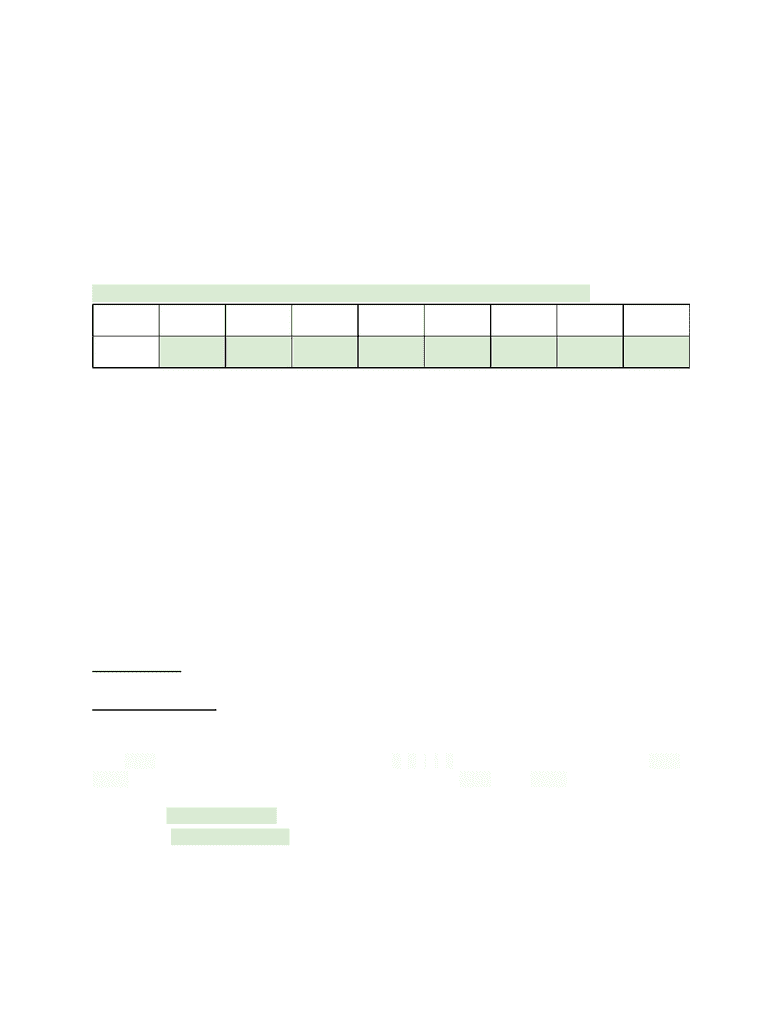Textbook Notes (280,000)
US (110,000)
Purdue (700)
CS (30)
Chapter 3

# CS 17700 Chapter Notes - Chapter 3: Empty String, Empty Spaces, Code Injection

Department
Computer Sciences
Course Code
CS 17700
Professor
Gary Mc Fall
Chapter
3

This preview shows page 1. to view the full 4 pages of the document.CH3 Notes- Computing with Numbers
Objectives
-review the features and limitations of numeric data types in python
-review the concepts of data type conversions
-practice and apply numeric data type principles
-understand and apply round methods
-explore binary numbers
Computers only store and access data in binary (converted into binary and decimal later)
Fill in the binary in the boxes, smallest to largest, fill empty spaces with zeros
Decimal
128
64
32
16
8
4
2
1
Bianary
0
1
0
1
1
0
1
1
(0 * 128) + (1* 64) + (0*32) + (16* 1) + (1* 8)+ (0*4)+ (2*1) + (1*1) = 91
Or: 64+16+8+2+1 = 91
Converting Binary to Decimal:
1. Place the number in between the appropriate values (24 is between 32 & 16)
2. Put a zero on the larger number (32) and subtract the smaller number from your
value (24-16 = 8)
a. If you have a number greater than zero place a 1 in the binary box
b. If your number is zero place a zero in the box and all the remaining boxes
(working left to right)
3. If a. Then move on to the next right number, subtract, and repeat this until you end
with a zero (warning if you get past “1” and you still have a remainder you’ve done
something wrong)
Numeric Data Types:
data= information stored and manipulated by computer programs
USE THIS---> Int: positive or negative whole numbers, no decimal places
Ex -10, and 100,000,000,000
BEFORE THIS---->Float: Numbers including decimal places
Ex. 3.14159, 1.0
String: A 'string' is simply a list of characters in order.
Ex. "hello" is a string. It is five characters long — h, e, l, l, o. Strings can also have spaces: "hello
world" contains 11 characters, including the space between "hello" and "world" or even 0
characters, which is usually called "the empty string."
>>> print("Hello, world!")
>>> print ("bouncy, " * 10)